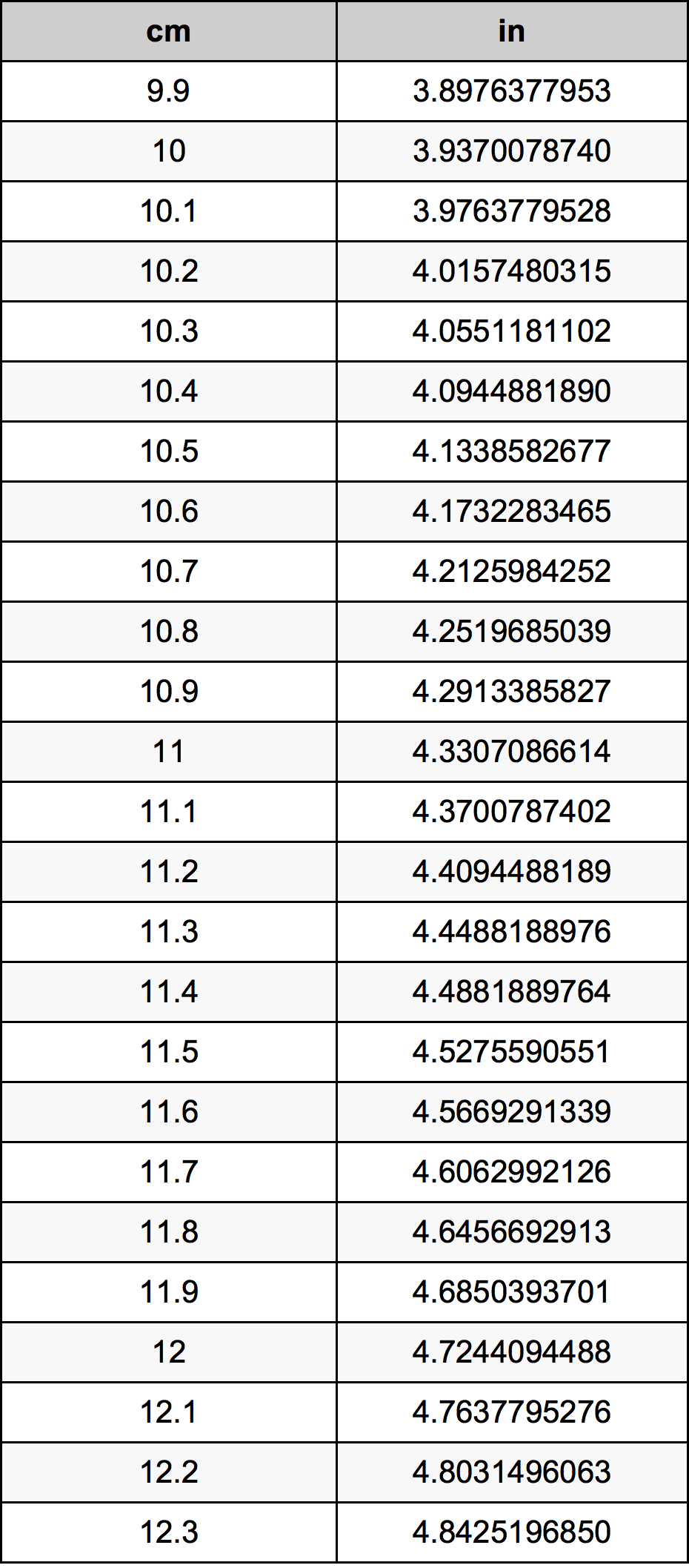Cm To Inches

# 11.1 cm to in11.1 Centimeters to Inches

cm
=
in

## How to convert 11.1 centimeters to inches?

 11.1 cm * 0.3937007874 in = 4.3700787402 in 1 cm
A common question is How many centimeter in 11.1 inch? And the answer is 28.194 cm in 11.1 in. Likewise the question how many inch in 11.1 centimeter has the answer of 4.3700787402 in in 11.1 cm.

## How much are 11.1 centimeters in inches?

11.1 centimeters equal 4.3700787402 inches (11.1cm = 4.3700787402in). Converting 11.1 cm to in is easy. Simply use our calculator above, or apply the formula to change the length 11.1 cm to in.

## Convert 11.1 cm to common lengths

UnitLength
Nanometer111000000.0 nm
Micrometer111000.0 µm
Millimeter111.0 mm
Centimeter11.1 cm
Inch4.3700787402 in
Foot0.3641732283 ft
Yard0.1213910761 yd
Meter0.111 m
Kilometer0.000111 km
Mile6.89722e-05 mi
Nautical mile5.99352e-05 nmi

## What is 11.1 centimeters in in?

To convert 11.1 cm to in multiply the length in centimeters by 0.3937007874. The 11.1 cm in in formula is [in] = 11.1 * 0.3937007874. Thus, for 11.1 centimeters in inch we get 4.3700787402 in.

## 11.1 Centimeter Conversion Table## Alternative spelling

11.1 Centimeter to Inch, 11.1 Centimeter in Inch, 11.1 Centimeters to in, 11.1 Centimeters in in, 11.1 cm to in, 11.1 cm in in, 11.1 Centimeter to in, 11.1 Centimeter in in, 11.1 cm to Inch, 11.1 cm in Inch, 11.1 Centimeter to Inches, 11.1 Centimeter in Inches, 11.1 Centimeters to Inches, 11.1 Centimeters in Inches